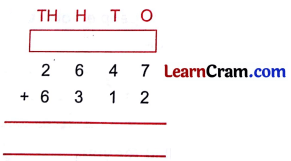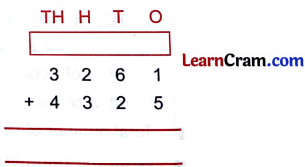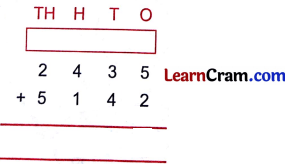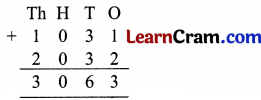# DAV Class 3 Maths Chapter 2 Worksheet 1 Solutions

The DAV Class 3 Maths Book Solutions and DAV Class 3 Maths Chapter 2 Worksheet 1 Solutions of Additions offer comprehensive answers to textbook questions.

## DAV Class 3 Maths Ch 2 WS 1 Solutions

1.Solution:
8955

2.Solution:
98673.Solution:
9898

4.Solution:
8959

5.Solution:
74376.Solution:
7586

7.Solution:
7577

8.Solution:
6999

9.Solution:
3866### DAV Class 3 Maths Chapter 2 Worksheet 1 Notes

1. The sum of numbers in any order is same.
1 + 3 + 4 = 8 or
4 + 3 + 1 = 8

2. Number + 0 = same number.
8 + 0 = 8
6 + 0 = 6
1378 + 0 = 1378

3. Addition of 10, 100 and 1000s
10 + 10 = 20
20 + 10 = 30
30 + 10 = 40
40 + 10 = 50
100 + 100 = 200
100 + 200 = 300
300 + 100 = 400
400 + 100 = 500
1000 + 1000 = 2000
2000 + 1000 = 3000
3000 + 1000 =4000
4000 + 1000 = 5000

1.Remember: First add ones, next tens, then hundreds, lastly thousands.

2.Question 1.
Collection of stamps of Rohan, Mohit, Renu and Neha are given below:
Mohit 159
Rohan 282
Renu 295
Neha 800

(a) Whoa has maximum number of stamps?
Solution:
Neha

(b) What is the total collections of stamps of Rohan and Renu?
Solution:
577

(c) How many stamps were collected by Neha and Mohit together?
Solution:
959

(d) If I give Rohan 18 stamps more, how many stamps will he be having now:
Solution:
300

Question 2.
A train was carrying 615 people. At a station 26 more people got into the train. Find the total number of people in the train now.
Solution:
No. of people in the train = 615
No. of people who got in = 26
Total = 641
Now, the total no. of people in the train is 641.Question 3.
Write the numbers on each petal. One has been filled for you.
Solution:
Do yourself.